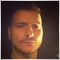# Sell RSI indicator by Howard Wang (as featured on the TASC Magazine in Feb 2019)16

Hello,

I was wondering if anyone could help me in coding this indicator, which is supposed to be better than the RSI. It was featured on the February issue of the "Technical Analysis of Stock and Commodities Magazine".

It can be found in these 2 places:

Thank you.16

For some reason my links were removed. So here it follows the code for EasyLanguage if a good soul want to translate it to MQL4 and/or MQL5:

Length( 20):
vars:
srsi(0) srsin(0),
k(0);
srsin =0;
For k =0 to Length-1
Begin
Value1 = absvalue(close[K]-open(k);
Value2 = absvalue(highk]-low(k));
If value2 = 0 then value3=0
Else
Value3 = valuel/value2;
srsin = srsin + value3;
End;
srsi = srsin/length;
plot1(0.38);
plot2(srsi);
plot3(-0.38);
plot40);
If srsi > O then Setplotcolor(2,green);
If srsi < O then Setplotcolor(2,red);
If srsi >=-0.05 and srsi <= 0.05 then Setplotcolor(2,yellow);
Setplotcolor(1,darkred);
Setplotcolor(3,darkgreen);
Setplotcolor(4,yellow);

Thank you.1235

There are some issues with this algorithm, maybe it's simple typos.

• srsi can never become negative, so why testing for srsi>=-0.05
• what means plot40) ?
• what is O in srsi > O
• does Length(20) mean it's fixed period or may that be user defined
• highk-lowk is always positive so why absvalue
Edit: I just looked up the original code, issues are clarified. absvalue needs to be removed entirely, O means zero.16

Nobody?23973

Try this :

```//+------------------------------------------------------------------+
//|                                                  MQLForumRSI.mq4 |
//|                              |
//+------------------------------------------------------------------+
#property version   "1.00"
#property strict
#property indicator_separate_window
#property indicator_maximum 0.618
#property indicator_minimum -0.618
#property indicator_buffers 3
#property indicator_plots   3
//--- plot base FRSI
#property indicator_label1  "base_FRSI"
#property indicator_type1   DRAW_LINE
#property indicator_color1  clrWhite
#property indicator_style1  STYLE_SOLID
#property indicator_width1  1
//--- plot overbought FRSI
#property indicator_label2  "ob_FRSI"
#property indicator_type2   DRAW_LINE
#property indicator_color2  clrOrangeRed
#property indicator_style2  STYLE_SOLID
#property indicator_width2  1
//--- plot oversold FRSI
#property indicator_label3  "os_FRSI"
#property indicator_type3   DRAW_LINE
#property indicator_color3  clrDodgerBlue
#property indicator_style3  STYLE_SOLID
#property indicator_width3  1
#property indicator_levelcolor clrYellow
#property indicator_level1 0.0
#property indicator_level2 0.38
#property indicator_level3 -0.38

input int RsiPeriod=21;//Period :
input double RsiOverbought=0.118;//Overbought
input double RsiOversold=-0.118;//Oversold

//--- indicator buffers
double         FRSIBuffer[];
double         obBuffer[];
double         osBuffer[];
//training #845
datetime barstamp=0;
bool FirstCalculation=false;
int bar_reduction=0;
//+------------------------------------------------------------------+
//| Custom indicator initialization function                         |
//+------------------------------------------------------------------+
int OnInit()
{
//--- indicator buffers mapping
SetIndexBuffer(0,FRSIBuffer);
SetIndexBuffer(1,obBuffer);
SetIndexBuffer(2,osBuffer);
FirstCalculation=false;
barstamp=0;
bar_reduction=RsiPeriod;

//---
return(INIT_SUCCEEDED);
}
//+------------------------------------------------------------------+
//| Custom indicator iteration function                              |
//+------------------------------------------------------------------+
int OnCalculate(const int rates_total,
const int prev_calculated,
const datetime &time[],
const double &open[],
const double &high[],
const double &low[],
const double &close[],
const long &tick_volume[],
const long &volume[],
{
//---
double this_item=0;
bool new_bar=false;
bool new_data=false;
int first_bar=1;
int last_bar=1;
if(Time!=barstamp)
{
new_bar=true;
barstamp=Time;
}
if(prev_calculated==0&&FirstCalculation==true) new_data=true;
if((prev_calculated==0&&FirstCalculation==false)||new_data==true) first_bar=rates_total-bar_reduction-1;
if(prev_calculated!=0&&FirstCalculation==true&&new_bar==true&&new_data==false) first_bar=1;
//Loop
for(int z=first_bar;z>=last_bar;z--)
{
int rsi_start=z+RsiPeriod;
int items=0;
double sum=0;
//inner loop
for(int i=rsi_start;i>=z;i--)
{
items++;
double value_1=Close[i]-Open[i];
double value_2=High[i]-Low[i];
this_item=0;
if(value_2>0) this_item=value_1/value_2;
sum+=this_item;
}
if(items>0)
{
sum=sum/items;
FRSIBuffer[z]=sum;
if(sum<=RsiOversold) osBuffer[z]=sum;
if(sum>=RsiOverbought) obBuffer[z]=sum;
}
//inner loop ends here
}
//Loop Ends Here
FirstCalculation=true;
//--- return value of prev_calculated for next call
return(rates_total);
}
```16

Lorentzos Roussos:

Try this :

Lorentzos, thank you for your help. I tried it on MT4 but it’s not working well if I scroll back a few days. Please take a look into the picture attached.
Files:16

DudaoRJ:
Lorentzos, thank you for your help. I tried it on MT4 but it’s not working well if I scroll back a few days. Please take a look into the picture attached.
Anyways, for visual testing purposes it’s working fine. In case of errors you just have to recalculate it. Thanks a lot.